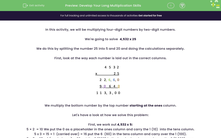# Develop Your Long Multiplication Skills

In this worksheet, students will learn how to multiply four-digit numbers by two-digit numbers.Key stage:  KS 2

Curriculum topic:   Number: Addition, Subtraction, Multiplication and Division

Curriculum subtopic:   Multiply to Four Digits (Long Multiplication)

Difficulty level:#### Worksheet Overview

In this activity, we will be multiplying four-digit numbers by two-digit numbers.

We're going to solve  4,532 x 25

We do this by splitting the number 25 into 5 and 20 and doing the calculations separately.

First, look at the way each number is laid out in the correct columns.We multiply the bottom number by the top number starting at the ones column.

Let's have a look at how we solve this problem:

First, we work out 4,532 x 5:

5 × 2  = 10 We put the 0 as a placeholder in the ones column and carry the 1 (10)  into the tens column.

5 x 3 = 15 + 1  (carried over) = 16 put the 6  (60) in the tens column and carry over the 1 (100).

5 × 5 = 25 + 1 (carried over) = 26 put the 6 (600) in the hundreds column and carry the 2 (2,000) over.

5 × 4 = 20 + 2 (carried over) = 22 put 2 (2,000) in the thousands column and the 2 (20,000) in the ten thousands column.

This tells us that 4,532 x 5 = 22,660

Now we move across to multiplying by 2 (20).

We start by putting a 0 as a placeholder in the ones column on the line below.

Now we carry on in the same way:

2 x 2 = 4

2 x 3 = 6

2 x 5 = 10 put the 0 as a placeholder and carry the 1 (10,000)

2 x 4 = 8 + 1 (carried over) = 9

4,532 x 20 = 90,640

Finally, add the two numbers together: 22,660  + 90,640 = 113,300

Phew! That was a lot to do!

Now, let's put this method to the test! You can look back at this introduction at any point by clicking on the red help button on the screen.### What is EdPlace?

We're your National Curriculum aligned online education content provider helping each child succeed in English, maths and science from year 1 to GCSE. With an EdPlace account you’ll be able to track and measure progress, helping each child achieve their best. We build confidence and attainment by personalising each child’s learning at a level that suits them.

Get started••••